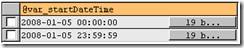If you ever have to do any ETL type work then at some point you’re probably going to have to work with data on a daily batch basis. Many people make use of the DATEPART function in T-SQL, or the DATE function in MySQL for this type of work. If you do something like…

TSQL

``````SELECT col1, col2, col3, col4
FROM myTable
WHERE DATEPART(yy, field) = 2009
AND DATEPART(mm, field) = 1
AND DATEPART(dd, field = 1;
``````

MySQL

``````SELECT col1, col2, col3, col4
FROM myTable
WHERE DATE(field) = ‘2008-01-01’;
``````

while this works, you will be unable to use an index on this column. You could get around this by adding a computed column, and then index that, but luckily there’s a simpler solution. Pre-calculate datetime ranges using variables then index use should be possible.

TSQL

``````DECLARE @startDateTime DATETIME,
@endDateTime DATETIME,
@date DATETIME;

SET @date = GETDATE();

SET @startDateTime = CAST(CAST(DATEPART(yyyy, @date) AS CHAR(4)) + '-' + CAST(DATEPART(mm, @date) AS CHAR(2)) + '-' + CAST(DATEPART(dd, @date) AS CHAR(2)) + ' 00:00:00' AS DATETIME);

SET @endDateTime = CAST(CAST(DATEPART(yyyy, @date) AS CHAR(4)) + '-' + CAST(DATEPART(mm, @date) AS CHAR(2)) + '-' + CAST(DATEPART(dd, @date) AS CHAR(2)) + ' 23:59:59' AS DATETIME);

SELECT @startDateTime;
SELECT @endDateTime;
``````

The above T-SQL will generate datetime values that will cover the whole of one day. You can then use the @startDateTime and @endDateTime variables to do;

``````SELECT col1, col2, col3, col4
FROM myTable
WHERE field BETWEEN @startDateTime AND @endDateTime
``````

Effectively you are saying “give me data for this whole day.”

MySQL

Below is the MySQL equivalent of the above wrapped up in a procedure.
``````DELIMITER \$\$

DROP PROCEDURE IF EXISTS `blog`.`usp_dateCalc`\$\$

CREATE PROCEDURE `blog`.`usp_dateCalc`(IN var_datetime DATETIME, OUT var_startDateTime DATETIME, OUT var_endDateTime DATETIME)
LANGUAGE SQL
SQL SECURITY INVOKER
COMMENT 'Calculating start and end datetimes for a date'
BEGIN

# Default to today if null
IF(var_datetime IS NULL) THEN
SET var_datetime = NOW();
END IF;

# Calculate the start and end of the day
SET var_startDateTime = CONCAT(DATE(var_datetime), ' 00:00:00');
SET var_endDateTime = CONCAT(DATE(var_datetime), ' 23:59:59');

END\$\$

DELIMITER ;
``````

This is how you use the procedure.

``````CALL usp_dateCalc('2008-01-05 09:30:30', @var_startDateTime, @var_endDateTime);
# Lets look at the variables
SELECT @var_startDateTime
UNION ALL
SELECT @var_endDateTime;
# Use these variables in our queries
SELECT col1, col2, col3, col4
FROM MyTable
WHERE field BETWEEN @var_startDateTime AND @var_endDateTime;
``````I’ve used this technique in daily ETL to take advantage of indexes on datetime columns. There’s no reason why you cannot use this idea for weekly, monthly or quarterly time periods and take advantage of those indexed datetime columns.